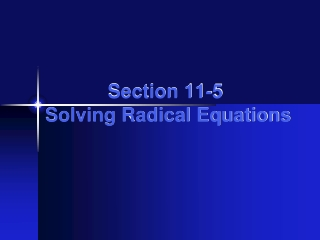DownloadDownload Presentation# Section 11-5 Solving Radical Equations

Télécharger la présentation## Section 11-5 Solving Radical Equations

- - - - - - - - - - - - - - - - - - - - - - - - - - - E N D - - - - - - - - - - - - - - - - - - - - - - - - - - -
##### Presentation Transcript

1. Section 11-5 Solving Radical Equations

2. Warm Up • Solve each equation. • 1. 3x +5 = 17 • 2. 4x + 1 = 2x – 3 • 3. • 4. (x + 7)(x – 4) = 0 • 5. x2 – 11x + 30 = 0 • 6.x2 = 2x + 15 4 –2 35 –7, 4 6, 5 5, –3

3. California Standards Extension of 2.0 Students understand and use such operations as taking the opposite, finding the reciprocal, taking a root, and raising to a fractional power. They understand and use the rules of exponents. Objectives

4. Vocabulary radical equation A radical equation is an equation that contains a variable within a radical.

5. Check 5  5 5 Solving Simple Radical Equations Solve the equation. Check your answer. Square both sides. x = 25 Substitute 25 for x in the original equation. Simplify.

6. Check 10 10 Solving Simple Radical Equations Solve the equation. Check your answer. Square both sides. 100 = 2x Divide both sides by 2. 50 = x 

7. Check Lets Try Together: Solve the equation. Check your answer. Square both sides. Simplify.  6 6

8. You try: Solve the equation. Check your answer. • 1) • 2) • 3) 3 = x x = 12

9. Solving Simple Radical Equations To solve these equations, you may have to isolate the square root +4 +4 Add 4 to both sides. Square both sides. x = 81

10. Solving Simple Radical Equations To solve these equations, you may have to isolate the square root Square both sides. Subtract 3 from both sides. -3 -3 x = 46

11. Lets try together: To solve these equations, you may have to isolate the square root Subtract 6 from both sides. -6 -6 Square both sides. Subtract 1 from both sides. 5x + 1 = 16 -1 -1 5x = 15 Divide both sides by 5. x = 3

12. You Try: Solving Simple Radical Equations To solve these equations, you may have to isolate the square root x = 9 x = 18 x = 3 1) 2) 3)

13. Solving Radical Equations by Multiplying or Dividing Method 1 Method 2 Square both sides. Divide both sides by 4. Square both sides. Divide both sides by 16. x = 64 x = 64

14. Solving Radical Equations by Multiplying or Dividing Method 1 Method 2 Multiply both sides by 2. Square both sides. Square both sides. 144 = x Multiply both sides by 4. 144 = x

15. You try 64 = x x = 100 1) 2) 3)

16. Solving Radical Equations with Square Roots on Both Sides Square both sides. 2x – 1 = x + 7 -x -x +1 +1 Add 1 to both sides and subtract x from both sides. x = 8

17. Add to both sides. Solving Radical Equations with Square Roots on Both Sides Square both sides. 5x – 4 = 6 Add 4 to both sides. +4 +4 5x = 10 x = 2

18. You try: x = 2 1) 2)

19. Lesson Quiz Solve each equation. Check your answer. 1. 2. 36 45 ø 3. 4. 11 5. 4 6. 4 7. A triangle has an area of 48 square feet, its base is 6 feet, and its height is feet. What is the value of x? What is the height of the triangle? 253; 16 ft

20. A triangle has an area of 36 square feet, its base is 8 feet, and its height is feet. What is the value of x? What is the height of the triangle? 8 ft Substitute 8 for b, 36 for A, and for h. : Geometry Application: Example 1 Use the formula for area of a triangle. Simplify. Divide both sides by 4.

21. A triangle has an area of 36 square feet, its base is 8 feet, and its height is feet. What is the value of x? What is the height of the triangle? 8 ft Example 1: Continued Square both sides. The value of x is 82. The height of the triangle is 9 feet. 81 = x – 1 82 = x

22. 5 Substitute 5 for w, 15 for A, and for l. Geometry Application: Example 2 A rectangle has an area of 15 cm2. Its width is 5 cm, and its length is ( ) cm. What is the value of x? What is the length of the rectangle? A = lw Use the formula for area of a rectangle. Divide both sides by 5.

23. Example 2: continued 5 The value of x is 8. The length of the rectangle is cm. A rectangle has an area of 15 cm2. Its width is 5 cm, and its length is ( ) cm. What is the value of x? What is the length of the rectangle? Square both sides. 9= x+1